Courses

# BJT Characteristics & Biasing - 2

## 15 Questions MCQ Test Topicwise Question Bank for Electrical Engineering | BJT Characteristics & Biasing - 2

Description
This mock test of BJT Characteristics & Biasing - 2 for Electrical Engineering (EE) helps you for every Electrical Engineering (EE) entrance exam. This contains 15 Multiple Choice Questions for Electrical Engineering (EE) BJT Characteristics & Biasing - 2 (mcq) to study with solutions a complete question bank. The solved questions answers in this BJT Characteristics & Biasing - 2 quiz give you a good mix of easy questions and tough questions. Electrical Engineering (EE) students definitely take this BJT Characteristics & Biasing - 2 exercise for a better result in the exam. You can find other BJT Characteristics & Biasing - 2 extra questions, long questions & short questions for Electrical Engineering (EE) on EduRev as well by searching above.
QUESTION: 1

Solution:
QUESTION: 2

### A device whose characteristics are very close to that of an ideal current source is

Solution:

The output characteristic of a CB transistor configuration is shown in figure below.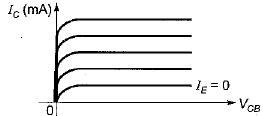It is clear that for a constant value of IE, IC is independent of VCB and the curves are parallel to the axis of VCB.
Hence, a CB transistor acts as an ideal current source.

QUESTION: 3

### The input resistance of a common-collector configuration will be of the order of

Solution:
QUESTION: 4

The common collector amplifier is also Known as

Solution:

A common collector amplifier is also known as Emitter follower because the output voltage (emitter voltage) will follow the input voltage.

QUESTION: 5

Consider the statements associated with various types of transistor configuration of a BJT:
1. In cut-off region, emitter and collector junctions are both reverse biased.
2. In saturation region, emitter junction is forward biased while collector junction can be either forward biased or reverse biased.
3. In active region, the collector junction is biased in the reverse biased and the emitter junction in the forward biased.
4. The phase shift introduced to the input in CC and CE configurations are each zero degree.

Q. Which of the above statements are correct ?

Solution:

• In saturation region, both emitter and collector junctions are forward biased.
• The phase shift introduced to the input signal in CC configuration is 0° while in CE configuration is 180°.
Hence, statements 2 and 4 are not correct.

QUESTION: 6

In an n-p-n transistor biased in the active region:

Solution:
QUESTION: 7

Assertion (A): An emitter follower is widely used in electronic instruments.
Reason (R): The voltage gain of emitter follower is very high.

Solution:

The voltage gain of emitter follower (or common collector configuration) is less than unity i.e. a low value.
Hence, reason is not true.

QUESTION: 8

Assertion (A): Common base amplifier has very low input resistance.
Reason (R): Low emitter ac resistance r'e shunts all other resistances.

Solution:
QUESTION: 9

Consider the following statements:
1. In a p-n-p transistor with normal bias, the collector junction is of low resistance.
2. p-n-p and n-p-n transistors can be interchanged with each other.
3. The circuit arrangement of a transistor used as a buffer is CC (Common Collector).
4. In a CE connected transistor, VCE will be equal to VCC when base current is zero.
5. In a transistor, emitter junction breakdown is due to “Zener effect” while collector junction breakdown is due to “Avalanche effect”.

Q. Which of the statements given above are not correct ?

Solution:

• For transistor operation, with normal bias, collector junction is reverse biased due to which collector junction is of high resistance.
• p-n-p and n-p-n transistors cannot he interchanged with each other.
• CC configuration has highest input resistance due to which it is used as buffer ampiifier for impedance matching.
• In CE transistor,
VCE = VCC - ICRC
When IB = 0,
IC is also equal to zero so that
VCE = VCC
• Breakdown voltage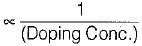Since emitter is heavily doped while collector is moderately doped, therefore, emitter junction breakdown is due to “Zener effect” while collector junction breakdown is due to “Avalanche effect.”
Thus, statements 1 and 2 are not correct.

QUESTION: 10

Match List-I with List-II and select the correct answer using the codes given below the lists:
List-I
A. Common emitter Configuration
B. Common collector Configuration
C. Base width modulation
D. Common base Configuration
List-II
1. Early Effect
2. Highest input resistance
3. Inverting amplifier
4. Lowest power gain
Codes: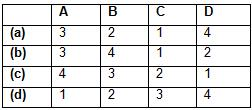Solution:
QUESTION: 11

For the transistor shown in the figure below, β = 25. The range of V1 such that, 1.0 < VCE  < 4.5 V is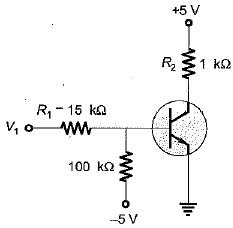Solution:

For VCE = 4.5 V,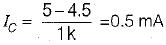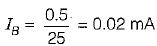R1 = 15 kΩ, R2 = 100 kΩ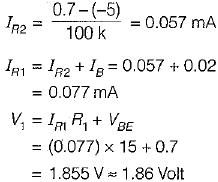For, VCE = 1.0 V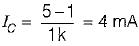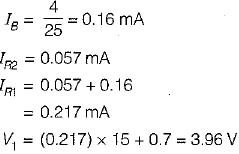So, 1.86 < V1 < 3.96

QUESTION: 12

In the bipolar source of figure the diode voltage and transistor VBE voltages are equal. If base current is neglected then collector current is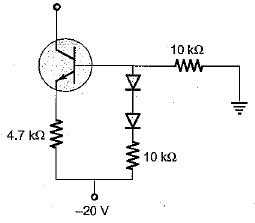Solution: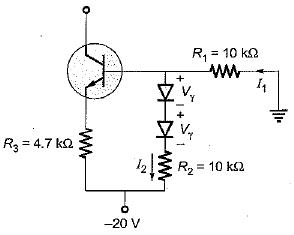Here,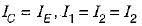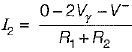or,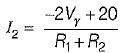..(i)
Applying KVL, we have: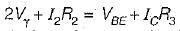..(ii)
Substituting I2 from equation (i) into equation (ii), we get: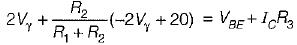Now,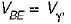R1 = R2 = 10 kΩ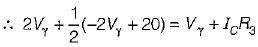or,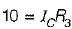or,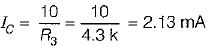∴ Collector current, IC = 2.13 mA

QUESTION: 13

The Early voltage of a BJT is VA = 75 volt. The minimum required collector current, such that the output resistance is atleast r0 = 200 KΩ is

Solution: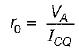or,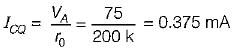QUESTION: 14

The thinnest region in a BJT is_____and the most doped region is________.

Solution:

Base is provided with smallest area to reduce transit type or journey time for the majority carriers while travelling from emitter to collector. Emitter is heavily doped to inject its majority carrier into base.

QUESTION: 15

In an n-p-n transistor biased in the active region, as the magnitude of the collector-base voltage is increased,

Solution:

IC increases because base width reduces due to early effect.